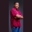Related Tags

array
swift
communitycreator

# How to find first array element satisfying a condition in SwiftTheodore Kelechukwu Onyejiaku

### Overview

We can find the first element of an array that satisfies a given condition using the first(where:) method.

### Syntax

array.first(where: condition)


### Parameters

array: This is the array whose elements we want to search to find the first element that satisfies a given condition.

condition: This is a closure that takes an array element as its argument and returns a Boolean value indicating whether there is an element that matches the condition.

### Complexity

$O(n)$. Here, n is the length of the array. This element that needs to be searched can be the last one of the array in the worst case.

### Return value

It is the first element of the array that satisfies the condition. Otherwise, null is returned if there is no element that satisfies the condition.

### Example

let evenNumbers = [2, 4, 6, 8, 10]
let twoLetterWords = ["up", "go", "me", "we"]
let numbers = [2, 3, -4, 5, -9]

// invoke the first(where:) method
let firstGreaterThanFive = evenNumbers.first(where: {$0 >= 5})! let firstTwoLetters = twoLetterWords.first(where: {$0.count == 2})!
let firstNegative = numbers.first(where: {\$0 < 0})!

// print results
print(firstGreaterThanFive)
print(firstTwoLetters)
print(firstNegative)
Returns first element that satisfies a condition

### Explanation

• Lines 1–3: We create three arrays.
• Lines 6–8: We call the first(where:) method on the arrays we created and store the returned results in some variables.
• Lines 11–13: We print the results.

RELATED TAGS

array
swift
communitycreator

CONTRIBUTORTheodore Kelechukwu Onyejiaku
RELATED COURSES

View all Courses

Keep Exploring

Learn in-demand tech skills in half the time